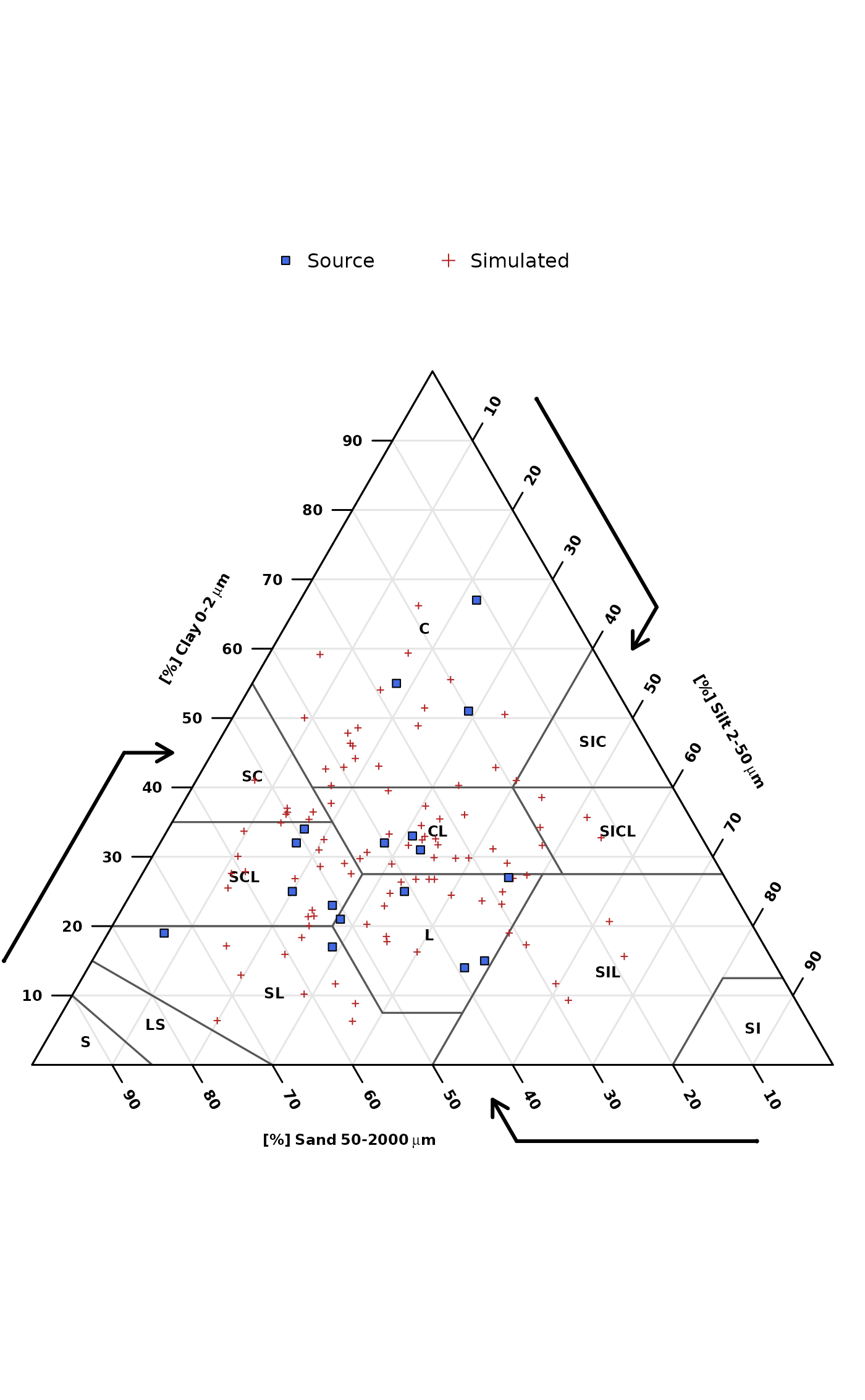Simulate realistic sand/silt/clay values (a composition) using multivariate Normal distribution or Dirichlet distribution. Simulations from the multivariate Normal distribution are based on the compositional mean and variance-covariance matrix. Simulations from the Dirichlet distribution are based on maximum likelihood estimation of alpha parameters.

bootstrapSoilTexture(ssc, method = c("dirichlet", "normal"), n = 100)

## Arguments

ssc

a data.frame object with 3 columns: sand, silt, clay and at least three rows of data within the range of 0-100 (percent). NA are automatically removed, but care should be taken to ensure that the sand/silt/clay values add to 100 percent. Simulations are based on these examples.

method

type of simulation: dirichlet or normal. See details.

n

number of simulated compositions. See details.

## Value

a list containing:

• samples - data.frame of simulated sand, silt, clay values

• mean - compositional mean

• var - compositional variance-covariance matrix

• D.alpha - (fitted) alpha parameters of the Dirichlet distribution, NULL when method = 'normal'

## Details

Simulations from the multivariate normal distribution will more closely track the marginal distributions of sand, silt, and clay--possibly a better fit for "squished" compositions (TODO elaborate). However, these simulations can result in extreme (unlikely) estimates.

Simulations from the Dirichlet distribution will usually be a better fit (fewer extreme estimates) but require a fairly large number of records in ssc (n >= 30?) for a reliable fit.

Additional examples will be added to this tutorial.

## Note

This is a work in progress.

D.E. Beaudette

## Examples


# \donttest{
if(
requireNamespace("compositions") &
requireNamespace("soiltexture")
) {

# sample data, data.frame
data('sp4')

# filter just Bt horizon data
ssc <- sp4[grep('^Bt', sp4$name), c('sand', 'silt', 'clay')] names(ssc) <- toupper(names(ssc)) # simulate 100 samples s <- bootstrapSoilTexture(ssc, n = 100) s <- s$samples

# empty soil texture triangle
TT <- soiltexture::TT.plot(
class.sys= "USDA-NCSS.TT",
main= "",
tri.sum.tst=FALSE,
cex.lab=0.75,
cex.axis=0.75,
frame.bg.col='white',
class.lab.col='black',
lwd.axis=1.5,
arrows.show=TRUE,
new.mar = c(3, 0, 0, 0)
)

# add original data points
soiltexture::TT.points(
tri.data = s, geo = TT, col='firebrick',
pch = 3, cex = 0.5, lwd = 1,
tri.sum.tst = FALSE
)

# add simulated points
soiltexture::TT.points(
tri.data = ssc, geo = TT, bg='royalblue',
pch = 22, cex = 1, lwd = 1,
tri.sum.tst = FALSE
)

# simple legend
legend('top',
legend = c('Source', 'Simulated'),
pch = c(22, 3),
col = c('black', 'firebrick'),
pt.bg = c('royalblue', NA),
horiz = TRUE, bty = 'n'
)

}